# AP Chemistry : Elemental Composition of Pure Substances

## Example Questions

← Previous 1 3 4 5 6 7

### Example Question #1 : Elemental Composition Of Pure Substances

What is the percent composition of potassium in K2Cr2O7?

26.5%

35.4%

22%

50%

26.5%

Explanation:

The molecular weight of K2Cr2O7 is 2(39 g/mol) + 2(52 g/mol) + 7(16 g/mol) = 294 g/mol.

Out of this total weight, the percent composition of K is: (2(39 g/mol)/294 g) = 26.5%

### Example Question #1 : Elemental Composition Of Pure Substances

Which of the following does not have the empirical formula CH?

C6H6

C100H100

Ethyne

C3H4

C3H4

Explanation:

An empirical formula represents a molecule with the simplest ratio. C6H6, ethyene (C2H2) and C100H100 can all be reduced to CH, however, C3H4 cannot because it does not have C and H atoms in a 1 : 1 ratio.

### Example Question #1 : Elemental Composition Of Pure Substances

An unknown compound is found to contain 37.47% carbon, 12.61% hydrogen, and 49.92% oxygen by mass. What is the empirical formula for this compound?

CH2O

C2H4O

C2H6O

CH4O

CH4O

Explanation:

If we assume that we have a sample of 100g of the compound, then that would mean we have 37.47 grams (3.12 moles) of carbon, 12.61 grams (12.48 moles) of hydrogen, and 49.92 grams (3.12 moles) of oxygen.

The molar ratio of C:H:O is 3:12:3, reducing to 1:4:1, giving the empirical formula of CH4O.

### Example Question #61 : Atomic Structure And Properties

What is the empirical formula for a sample that is 75% carbon by weight and 25% hydrogen by weight? (Note that this compound may not be realistic)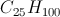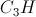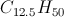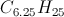Explanation:

To solve this problem, we will consider a sample that is 100g. We can assume that 75g of that sample is carbon and 25g of that sample is hydrogen.

First, we will determine the molar ratio of carbon to hydrogen.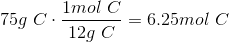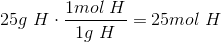Now we will determine the whole number ratio.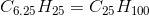### Example Question #2 : Elemental Composition Of Pure Substances

Below are the data for ionization energies of three elements X, Y, and Z. These elements are in the third period of the periodic table. The first four ionization energies for elements X, Y, and Z are given below in values of kJ/mol.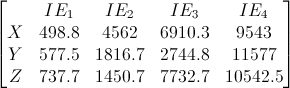Figure 1: Ionization energies in kJ/mol for selected elements.

If element Y formed a metal oxide, what would the molecuar formula be?

Please note that element Y is an element from the third period. It is NOT yttrium (Y).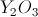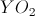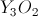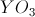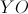Explanation:

The correct answer is. We found that element Y is aluminum due to its discrepancy between IE3 and IE4. Aluminum contains three valence electrons, and it needs to shed all three to reach a noble gas configuration. This makes it Al(III), or Al3+. Oxygen must gain two electrons to achieve a noble gas configuration. This makes it O2-. The only way to balance the opposing charges of the aluminum oxide is to have two aluminum ionically bonded to three oxygen.

Aluminum: charge +3; Oxygen charge: -2

2(+3) + 3(-2) = 0

### Example Question #1 : Elemental Composition Of Pure Substances

What is the empirical formula for a compound that is composed of 22.6% carbon, 1.9% hydrogen, and 75.5% oxygen?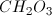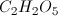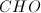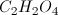Explanation:

When finding empirical formulas, take the percentages of each atom and convert them to grams by imagining a 100-gram sample of the compound.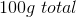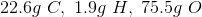Next, divide each mass by the molar mass of the atom.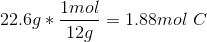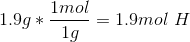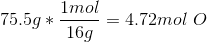Finally, divide each number by the smallest number out of the three. Remember that ratios must be a whole number.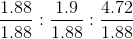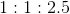Since dividing the number for oxygen by the smallest number gives a value of 2.5, we must multiply each atom by 2 in the empirical formula.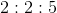This results in an empirical formula of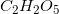.

### Example Question #61 : Atomic Structure And Properties

A mystery carbohydrate is composed of 25% carbon, 8.3% hydrogen, and 66.7% oxygen. What is the molecular formula of this mystery carbohydrate, assuming the molar mass of the compound is three times as heavy as the molar mass of the empirical formula?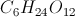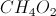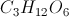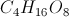Explanation:

In order to find the molecular formula, we must first find the empirical formula. We start by imagining a sample of the compound weighing 100 grams, so the percentages can be seen as grams. 25g of the sample is carbon, 8.3g of the sample is hydrogen, and 66.7g of the sample is oxygen.

Then, each of the atoms' masses is divided by the atom's molar mass, the numbers are then compared to each other in order to find the ratio of atoms.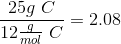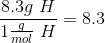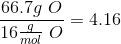There is a ratio of two carbon to eight hydrogen to four oxygen, which reduces to a 1:4:2 ratio between carbon, hydrogen, and oxygen respectively.

As a result, the empirical formula is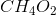. Since the molecular formula is three times as heavy, we simply multiply each portion of the empirical formula by three. The molecular formula is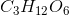.

### Example Question #741 : Ap Chemistry

What is the percent of carbon by mass in acetone CH3C(O)CH3?

10%

50%

62%

25%

75%

62%

Explanation:

To calculate the percent mass of a particular element within a substance I like to use a formulaic approach.

First multiply the number of moles of the atom of interest (C) by its molar mass.

3moles* 12 g/mol= 36g

Then divide by the overall molar mass of the molecule, obtain this by adding the atomic weights of each atom present in the molecule:

36g/58g= 62%

### Example Question #1 : Elemental Composition Of Pure Substances

What is the mass percentage of oxygen in aluminum (III) hydroxide?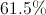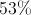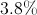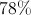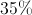Explanation:

The formula for aluminum hydroxide is: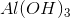To find the mass percentage, divide the total molar mass of oxygen by the formula mass of aluminum hydroxide.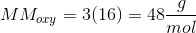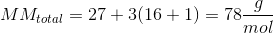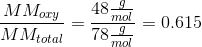Convert the ratio to a percentage to get our final answer.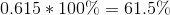### Example Question #3 : Elemental Composition Of Pure Substances

Suppose the mass of an unidentified substance is determined to be 53.3% carbon, 11.1% hydrogen, and 35.6% oxygen. It is also discovered that the number of moles of this substance in a 100 gram sample is 1.1 moles. What is the empirical formula for this substance? What is the molecular formula?

Empirical formula: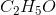Molecular formula: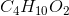Empirical formula:Molecular formula:Empirical formula:Molecular formula:Empirical formula: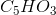Molecular formula: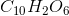Empirical formula:Molecular formula:Empirical formula:Molecular formula:Explanation:

To find the empirical formula, we have to determine a mole ratio of the elements in the substance, which we can get by dividing the mass percents of the elements by their respective atomic masses. Approximating: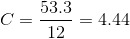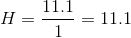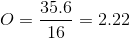To get an empirical formula we want to have whole number ratios, so we have to find a common whole number multiple or dividend that will make all of these pieces whole. A common strategy is to divide everything by the smallest number (in this case, 2.22).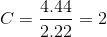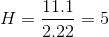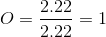We can now write our empirical formula using the above ratio for our subscripts:To find the molecular formula, we need to use the molar mass. We are given that 100 grams of this substance is equal to 1.1 moles; dividing grams by moles gives a molar mass of 91 grams per mole.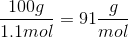The molar mass of is 45 grams per mole.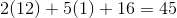We divide the molar mass of the actual substance by the molar mass of the empirical formula: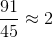Hence, 2 is the constant multiplier we apply to every subscript in our empirical formula, obtaining the final molecular formula:← Previous 1 3 4 5 6 7

### All AP Chemistry Resources